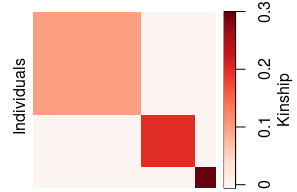# Simulate Admixed Populations with bnpsd

#### 2019-05-15

$\newcommand{\Fst}{F_{\text{ST}}} \newcommand{\ft}[j]{f_{#1}^T}$

# Introduction

The bnpsd package simulates the genotypes of an admixed population. In the PSD model (Pritchard, Stephens, and Donnelly 2000), admixed individuals draw their alleles with individual-specific probabilities (admixture proportions) from $$K$$ intermediate subpopulations. We impose the BN model (Balding and Nichols 1995) to the intermediate subpopulation allele frequency, which thus evolve independently with subpopulation-specific inbreeding coefficients ($$\Fst$$ values) from a common ancestral population $$T$$. The kinship coefficients and generalized $$\Fst$$ of the admixed individuals were derived in recent work (Ochoa and Storey 2016a). A simulated admixed population generated using this package was used to benchmark kinship and $$\Fst$$ estimators in the accompanying paper (Ochoa and Storey 2016b). Here we briefly summarize the notation and intuition behind the key parameters (see (Ochoa and Storey 2016a) for precise definitions).

There are conceptually two parts of the BN-PSD model, one that defines relatedness between individuals (under the admixture framework), while the other simulates allele frequencies and genotypes conditional on the specified parameters of relatedness/admixture.

## The BN-PSD population structure

The population structure determines how individuals are related to each other. The key parameters are the coancestry coefficients of the intermediate subpopulations and the admixture proportions of each individual for each subpopulation ($$q_{ju}$$), which are treated as fixed variables.

The bnpsd functions that model population structure (not drawing allele frequencies or genotypes) admit arbitrary coancestry matrices for the intermediate subpopulations. However, the code that draws allele frequencies and genotypes requires these subpopulations to be independent, which means that the coancestry matrix must have zero values off-diagonal. In this setting—the only case we’ll considered in this vignette—the diagonal values of the coancestry matrix of the intermediate subpopulations are inbreeding coefficients ($$\ft[S_u]$$ below), which correspond to subpopulation-specific $$\Fst$$ values.

Each intermediate subpopulation $$S_u$$ ($$u \in \{1, ..., K\}$$) evolved independently from a shared ancestral population $$T$$ with an inbreeding coefficient denoted by $$\ft[S_u]$$. Note that $$T$$ is a superscript, and does not stand for exponentiation or matrix transposition. Each admixed individual $$j \in \{1, ..., n\}$$ draws each allele from $$S_u$$ with probability given by the admixture proportion $$q_{ju}$$ ($$\sum_{u=1}^K q_{ju} = 1 \forall j$$). In this case the coancestry coefficients $$\theta^T_{jk}$$ between individuals $$j,k$$ (including $$j=k$$ case) and the $$\Fst$$ of the admixed individuals are given by: $\theta^T_{jk} = \sum_{u=1}^K q_{ju} q_{ku} \ft[S_u], \quad \quad \Fst = \sum_{j=1}^n \sum_{u=1}^K w_j q_{ju}^2 \ft[S_u],$ where $$0 < w_j < 1, \sum_{j=1}^n w_j = 1$$ are user-defined weights for individuals (default $$w_j=\frac{1}{n} \forall j$$). Note $$\theta^T_{jk}$$ equals the kinship coefficient for $$j \ne k$$ and the inbreeding coefficient for $$j=k$$.

The bias coefficient $$s$$ is defined by $s = \frac{\bar{\theta}^T}{\Fst}$ where $$\bar{\theta}^T = \sum_{j=1}^n \sum_{k=1}^n w_j w_k \theta_{jk}^T$$. This $$0 < s \le 1$$ approximates the proportional bias of $$\Fst$$ estimators that assume independent subpopulations, and one bnpsd function below fits its parameters to yield a desired $$s$$.

## Random allele frequencies and genotypes

This section details the distributions of the allele frequencies and genotypes of the various populations or individuals of the BN-PSD model.

Every biallelic locus $$i$$ in the ancestral population $$T$$ has an ancestral reference allele frequency denoted by $$p_i^T$$. By default the bnpsd code draws $p_i^T \sim \text{Uniform}(a, b)$ with $$a=0.01, b=0.5$$, but the code accepts $$p_i^T$$ from arbitrary distributions (see below).

The distribution of the allele frequency at locus $$i$$ in subpopulation $$S_u$$, denoted by $$p_i^{S_u}$$, is the BN distribution: $p_i^{S_u} | T \sim \text{Beta} \left( \nu_s p_i^T, \nu_s \left( 1-p_i^T \right) \right),$ where $$\nu_s = \frac{1}{\ft[S_u]}-1$$. Allele frequencies for different loci and different subpopulations ($$S_u,S_v, u \ne v$$) are drawn independently.

Each admixed individual $$j$$ at each locus $$i$$ draws alleles from a mixture of Bernoulli distributions from each intermediate subpopulation, which is mathematically equivalent to assigning what we call individual-specific allele frequencies $$\pi_{ij}$$ constructed as: $\pi_{ij} = \sum_{u=1}^K p_i^{S_u} q_{ju}.$ The unphased genotype $$x_{ij}$$ (encoded to count the number of reference alleles) is drawn as: $x_{ij}|\pi_{ij} \sim \text{Binomial}(2, \pi_{ij}).$

# Simulation examples

## Population structure: 1D geography

Let’s generate the same population structure used in the simulation of (Ochoa and Storey 2016b).

# this package
library(bnpsd)
# for nice colors
library(RColorBrewer)
# for visualizing coancestry matrix with plot_popkin, and other handy functions
library(popkin)

# dimensions of data/model
# number of individuals (NOTE this is 10x less than in publication!)
n_ind <- 100
# number of intermediate subpops
k_subpops <- 10

# define population structure
# subpopulation FST vector, up to a scalar
inbr_subpops <- 1 : k_subpops
# desired bias coefficient
bias_coeff <- 0.5
# desired FST for the admixed individuals
Fst <- 0.1
# admixture proportions from 1D geography
obj <- admix_prop_1d_linear(
n_ind = n_ind,
k_subpops = k_subpops,
bias_coeff = bias_coeff,
coanc_subpops = inbr_subpops,
fst = Fst
)
admix_proportions <- obj$admix_proportions # rescaled inbreeding vector for intermediate subpopulations inbr_subpops <- obj$coanc_subpops

# get pop structure parameters of the admixed individuals
coancestry <- coanc_admix(admix_proportions, inbr_subpops)

# verify that we got the desired FST!
fst_admix(admix_proportions, inbr_subpops)
#>  0.1

# the mean of the diagonal of the coancestry matrix also equals FST
inbr <- diag(coancestry)
fst(inbr) # fst is a function in the popkin package
#>  0.1

# verify that we got the desired bias_coeff too!
mean(coancestry) / Fst
#>  0.5
# visualize the per-subpopulation inbreeding coefficients (FSTs)
# shrink default margins
par(mar = c(4, 4, 0, 0) + 0.2)
# colors for independent subpopulations
col_subpops <- brewer.pal(k_subpops, "Paired")

barplot(inbr_subpops, col = col_subpops, names.arg = 1 : k_subpops, ylim = c(0, 1),
xlab = 'Subpopulation', ylab = 'Inbreeding coeff.')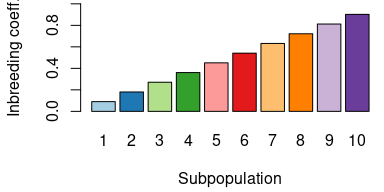# visualize the admixture proportions
# shrink default margins
par(mar = c(1, 4, 0, 0) + 0.2)
barplot(t(admix_proportions), col = col_subpops, border = NA, space = 0, ylab = 'Admixture prop.')
mtext('Individuals', 1)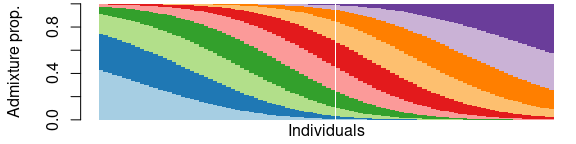# Visualize the coancestry matrix using "popkin"!
# set outer margin for axis labels (left and right are non-zero)
par(oma = c(0, 1.5, 0, 3))
# zero inner margin (plus padding) because we have no individual or subpopulation labels
par(mar = c(0, 0, 0, 0) + 0.2)
# now plot!
plot_popkin(coancestry)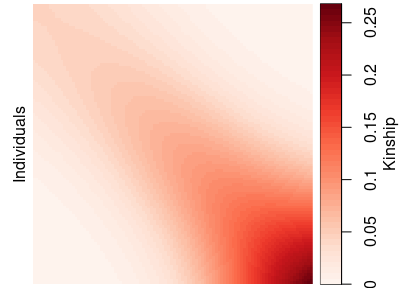## Draw random allele frequencies and genotypes

Now let’s draw all the random allele frequencies and genotypes from the population structure. The easiest way is to use draw_all_admix:

# number of loci in simulation (NOTE this is 30x less than in publication!)
m_loci <- 10000
# draw all random Allele Freqs (AFs) and genotypes
# reuse the previous inbr_subpops, admix_proportions
out <- draw_all_admix(
admix_proportions = admix_proportions,
inbr_subpops = inbr_subpops,
m_loci = m_loci,
# NOTE by default p_subpops and p_ind are not returned, but here we will ask for them
want_p_subpops = TRUE,
want_p_ind = TRUE
)
# genotypes
X <- out$X # ancestral AFs p_anc <- out$p_anc
# intermediate independent subpopulation AFs
p_subpops <- out$p_subpops # individual-specific AFs p_ind <- out$p_ind
# inspect distribution of ancestral AFs (~ Uniform(0.01, 0.5))
# shrink default margins for these figures
par(mar = c(4, 4, 0, 0) + 0.2)
hist(p_anc, xlab = 'Ancestral AF', main = '', xlim = c(0, 1))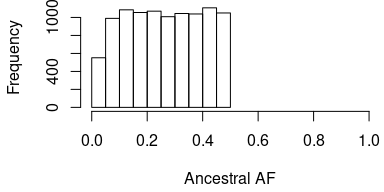# distribution of intermediate population AFs
# (all subpopulations combined)
# (will be more dispersed toward 0 and 1 than ancestral AFs)
hist(p_subpops, xlab = 'Intermediate Subpopulation AF', main = '', xlim = c(0, 1))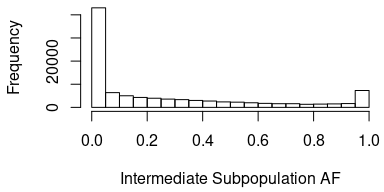# distribution of individual-specific AFs (admixed individuals)
# (admixture reduces differentiation, so these resemble ancestral AFs a bit more)
hist(p_ind, xlab = 'Individual-specific AF', main = '', xlim = c(0, 1))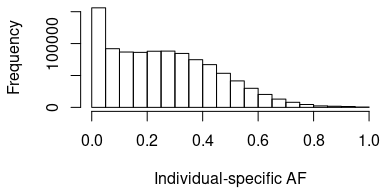# genotype distribution of admixed individuals
barplot(table(X), xlab = 'Genotypes', ylab = 'Frequency', col = 'white')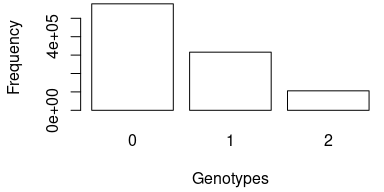Lastly, let’s verify that the correlation structure of the genotypes matches the theoretical coancestry matrix we constructed earlier. For this we use the popkin function of the package with the same name.

# for best estimates, group individuals into subpopulations using the geography
# this averages more individuals in estimating the minimum kinship
subpops <- ceiling( ( 1 : n_ind ) / n_ind * k_subpops )
table(subpops) # got k_subpops = 10 with 100 individuals each
#> subpops
#>  1  2  3  4  5  6  7  8  9 10
#> 10 10 10 10 10 10 10 10 10 10
# now estimate kinship using popkin
kinship_estimate <- popkin(X, subpops)
# replace diagonal with inbreeding coeffs. to match coancestry matrix
coancestry_estimate <- inbr_diag(kinship_estimate)

# Visualize the coancestry matrix using "popkin"!
# set outer margin for axis labels (left and right are non-zero)
par(oma = c(0, 1.5, 0, 3))
# increase inner top margin for panel titles
par(mar = c(0, 0, 2.5, 0) + 0.2)
# now plot!
coancestries <- list(coancestry, coancestry_estimate)
titles <- c('Truth', 'Estimate')
plot_popkin(coancestries, titles)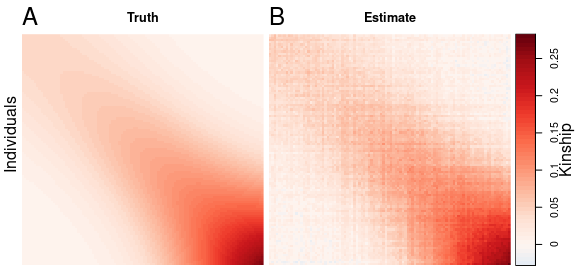## Customizing the allele frequency and genotype pipeline

The random variables generated by draw_all_admix above can also be generated separately using the following functions (where $$p$$ is the usual variable symbol for allele frequencies):

• draw_p_anc (drawn ancestral allele frequencies)
• draw_p_subpops (draw independent subpopulation allele frequencies)
• make_p_ind_admix (construct individual-specific allele frequencies under the admixture model)
• draw_genotypes_admix (draw genotypes under the admixture model)

These functions are provided for greater flexibility (examples follow further below). However, the joint function draw_all_admix has the advantage of removing fixed loci (loci that were randomly set to 0 or 2 for all individuals, despite non-zero allele frequencies), which are re-drawn from scratch (starting from the ancestral allele frequencies).

Here is the step-by-step procedure for drawing AFs and genotypes in the default draw_all_admix (except for the re-drawing of fixed loci):

# reuse the previous m_loci, inbr_subpops, admix_proportions
# draw ancestral AFs
p_anc <- draw_p_anc(m_loci)
# draw intermediate independent subpopulations AFs
p_subpops <- draw_p_subpops(p_anc, inbr_subpops)
# draw individual-specific AFs
p_ind <- make_p_ind_admix(p_subpops, admix_proportions)
# draw genotypes
X <- draw_genotypes_admix(p_ind)

We provide functions for these separate steps to allow for more flexibility. For example, the ancestral allele frequencies could be drawn from a Symmetric Beta instead of using draw_p_anc:

# this increases the proportion of rare alleles
alpha <- 1/2
p_anc <- rbeta(m_loci, alpha, alpha)
# shrink default margins for these figures
par(mar = c(4, 4, 0, 0) + 0.2)
hist(p_anc, xlab = 'Ancestral AF', main = '', xlim = c(0, 1))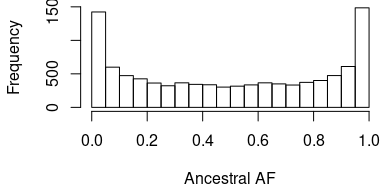You could also draw genotypes from the ancestral population or the intermediate populations:

# draw genotypes for one individual from the ancestral population
# use "cbind" to turn the vector p_anc into a column matrix
# ("draw_genotypes_admix" expects a matrix)
X_anc <- draw_genotypes_admix( cbind(p_anc) )
# returns a column matrix:
dim(X_anc)
#>  10000     1

# draw genotypes from intermediate populations
# draws one individual per intermediate population
X_subpops <- draw_genotypes_admix(p_subpops)

## Low-memory genotype simulation algorithm

If you desire to simulate a very large number of individuals ($$n$$) and loci ($$m$$), you might run out of memory while running draw_all_admix. Memory consumption is reduced by passing the low_mem = TRUE option to draw_all_admix, which draws the genotypes X directly from p_subpops and admix_proportions, without storing the entire intermediate matrix p_ind in memory at once. However, this algorithm can be much slower than the default one (low_mem = FALSE).

out <- draw_all_admix(admix_proportions, inbr_subpops, m_loci, low_mem = TRUE)
# genotypes
X <- out$X # ancestral AFs p_anc <- out$p_anc
# out$p_subpops missing (want_p_subpops = FALSE by default) # out$p_ind is not computed when low_mem = TRUE!

The same option exists for draw_genotypes_admix, which instead of accepting the p_ind matrix as input requires both p_subpops and admix_proportions as above:

X <- draw_genotypes_admix(p_subpops, admix_proportions, low_mem = TRUE)

# Additional population structures

Here we show examples for functions that create admixture matrices for various simple population structures. The admixture scenarios implemented in bnpsd are generated by these functions:

• admix_prop_1d_linear: Linear 1D geography
• admix_prop_1d_circular: Circular 1D geography
• admix_prop_indep_subpops: Independent Subpopulations

The main example (above) was for admix_prop_1d_linear, the rest follow.

## Circular 1D geography

This is a twist on the earlier 1D geography where subpopulations and individuals are placed on a circumference, so random walks wrap around and the appropriate density is the Von Misses distribution.

Let’s generate an analogous population structure to the original “linear” example.

# data dimensions
# number of individuals
n_ind <- 100
# number of intermediate subpops
k_subpops <- 10

# define population structure
# subpopulation FST vector, up to a scalar
inbr_subpops <- 1 : k_subpops
# desired bias coefficient
bias_coeff <- 0.5
# desired FST for the admixed individuals
Fst <- 0.1

# admixture proportions from *circular* 1D geography
obj <- admix_prop_1d_circular(
n_ind = n_ind,
k_subpops = k_subpops,
bias_coeff = bias_coeff,
coanc_subpops = inbr_subpops,
fst = Fst
)
admix_proportions <- obj$admix_proportions inbr_subpops <- obj$coanc_subpops

# get pop structure parameters of the admixed individuals
coancestry <- coanc_admix(admix_proportions, inbr_subpops)

# verify that we got the desired FST!
fst_admix(admix_proportions, inbr_subpops)
#>  0.1

# verify that we got the desired bias_coeff too!
mean(coancestry) / Fst
#>  0.5
# visualize the per-subpopulation inbreeding coefficients (FSTs)
# tweak margins/etc
par(mar = c(2.5, 2.5, 0.3, 0) + 0.2, lab = c(2, 1, 7), mgp = c(1.5, 0.5, 0))
# colors for independent subpopulations
col_subpops <- brewer.pal(k_subpops, "Paired")
barplot(inbr_subpops, col = col_subpops, names.arg = colnames(admix_proportions), xlab = 'Subpopulation', ylab = 'Inbr')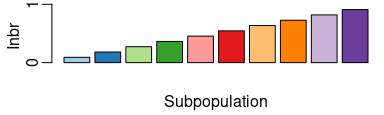# visualize the admixture proportions
# tweak margins/etc
par(mar = c(1, 4, 0.4, 0) + 0.2, lab = c(2, 2, 7))
barplot(t(admix_proportions), col = col_subpops, border = NA, space = 0, ylab = 'Admix prop')
mtext('Individuals', 1)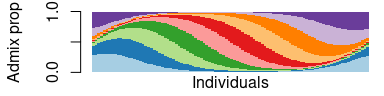# Visualize the coancestry matrix using "popkin"!
# tweak margins/etc
par(oma = c(0, 1.5, 0, 3), mar = c(0, 0, 0.4, 0) + 0.2)
plot_popkin(coancestry, leg_n = 3)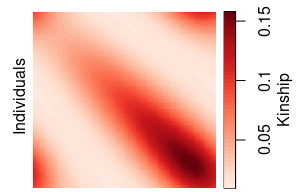## Independent subpopulations

The independent subpopulations model, where individuals are actually unadmixed, is the most trivial form of the BN-PSD admixture model.

# define population structure
# we'll have k_subpops = 3, each subpopulation with these sizes:
n1 <- 100
n2 <- 50
n3 <- 20

# here's the labels (for simplicity, list all individuals of S1 first, then S2, then S3)
labs <- c(
rep.int('S1', n1),
rep.int('S2', n2),
rep.int('S3', n3)
)
# data dimensions infered from labs:

# number of individuals "n_ind"
length(labs)
#>  170

# number of subpopulations "k_subpops"
k_subpops <- length(unique(labs))
k_subpops
#>  3

# desired admixture matrix
admix_proportions <- admix_prop_indep_subpops(labs)

# got a numeric matrix with a single 1 value per row
# (denoting the sole subpopulation from which each individual draws its ancestry)
head(admix_proportions, 2)
#>      S1 S2 S3
#> [1,]  1  0  0
#> [2,]  1  0  0

# construct the intermediate subpopulation FST vector
# the desired final FST
Fst <- 0.2
# subpopulation FST vector, unnormalized so far
inbr_subpops <- 1 : k_subpops
# normalized to have the desired FST
# NOTE fst is a function in the popkin package
inbr_subpops <- inbr_subpops / fst(inbr_subpops) * Fst
# verify FST for the intermediate subpopulations
fst(inbr_subpops)
#>  0.2

# get coancestry of the admixed individuals
coancestry <- coanc_admix(admix_proportions, inbr_subpops)
# before getting FST for individuals, weigh then inversely proportional to subpop sizes
weights <- weights_subpops(labs) # function from popkin package
# verify FST for individuals (same as for intermediate subpops for this pop structure)
fst_admix(admix_proportions, inbr_subpops, weights)
#>  0.2
# visualize the per-subpopulation inbreeding coefficients (FSTs)
# tweak margins/etc
par(mar = c(2.5, 2.5, 0, 0) + 0.2, lab = c(2, 1, 7), mgp = c(1.5, 0.5, 0))
# colors for independent subpopulations
col_subpops <- brewer.pal(k_subpops, "Paired")
barplot(inbr_subpops, col = col_subpops, names.arg = colnames(admix_proportions), xlab = 'Subpopulation', ylab = 'Inbr')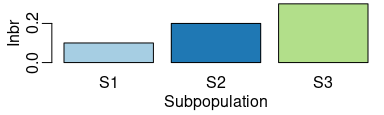# visualize the admixture proportions
# tweak margins/etc
par(mar = c(1, 4, 0.4, 0) + 0.2, lab = c(2, 2, 7))
barplot(t(admix_proportions), col = col_subpops, border = NA, space = 0, ylab = 'Admix prop')
mtext('Individuals', 1)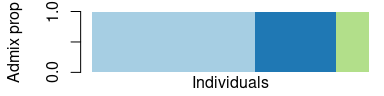# Visualize the coancestry matrix using "popkin"!
# tweak margins/etc
par(oma = c(0, 1.5, 0, 3), mar = c(0, 0, 0.4, 0) + 0.2)
plot_popkin(coancestry, leg_n = 3)